# ASMSchool - Lesson 2

## Arithmetic and Logical Operators!

Here's where things get fun! Now that we know how to move data around to suit our needs, we need to know how to MANIPULATE data! We want to mess with it, we want to change it, we want to screw it all up! And this is where all that happens. Most of the arithmetic and logical operations can only be performed between the accumulator and either an 8-bit register, a byte of immediate data, or a byte of data located in memory that is indexed by register pair HL. Note that arithmetic and logical operators DO NOT allow direct addressing. If an operation produces a result, it replaces the operand in A with the result. Note that some instructions don't have direct results, some just affect flags in the F register.

Ok, first on our list of math is the 8-bit Arithmetic and Logical opcodes. These work with 8-bit pieces of data and produce results, if any, that are 8-bit. Of course, almost every instruction affects one of the four flags in the F register, and the flags affected are listed with each instruction.

## 8-bit Arithmetic and Logical Instructions

This is the very simple ADD instruction. It basically adds the contents of register A with the second operand, and places the result in A. The second operand can be either an 8-bit register r, or a single byte of immediate data n, or a byte in memory addressed by the register pair HL.
Flags affected are:
Z: set according to result
C: set according to result
N: reset (0)
H: set according to result

This is the ADD with Carry instruction. It acts exactly like a standard ADD, including operands, except that it adds the contents of the single-bit Carry flag to the result. Helpful for things like adding large numbers. =)
Z: set according to result
C: set according to result
N: reset (0)
H: set according to result

SUB r
SUB n
SUB (HL)

The SUB instruction subtracts the operand from the A register and leaves the result in A. Same types of operands as the ADD instruction.
Z: set according to result
C: set according to result
N: set (1)
H: set according to result

NOTE: this instruction DOES NOT have a destination specified because it is implied as ALWAYS being register A.

SBC A, r
SBC A, n
SBC A, (HL)

This is (as you probably guessed) the SUB instruction with Carry. It subtracts the Carry flag from the result.
Z: set according to result
C: set according to result
N: set (1)
H: set according to result

AND r
AND n
AND (HL)

This instruction LOGICALLY AND's each bit of both register A and the operand together and leaves the result in A.
Z: set according to result
C: reset (0)
N: reset (0)
H: set (1)

NOTE: this instruction DOES NOT have a destination specified because it is implied as ALWAYS being register A.

OR r
OR n
OR (HL)

This instruction LOGICALLY OR's each bit of both register A and the operand together and leaves the result in A.
Z: set according to result
C: reset (0)
N: reset (0)
H: reset (0)

NOTE: this instruction DOES NOT have a destination specified because it is implied as ALWAYS being register A.

XOR r
XOR n
XOR (HL)

This instruction LOGICALLY XOR's each bit of both register A and the operand together and leaves the result in A.
Z: set according to result
C: reset (0)
N: reset (0)
H: reset (0)

NOTE: this instruction DOES NOT have a destination specified because it is implied as ALWAYS being register A.

CP r
CP n
CP (HL)
This instruction ComPares the operand with the contents of the accumulator by subtracting the operand from the accumulator. This instruction differs from the SUB instruction in that the contents of A aren't changed, only the flags.
Z: set according to result
C: set according to result
N: set (1)
H: set according to result

NOTE: this instruction DOES NOT have a destination specified because it is implied as ALWAYS being register A.

INC r
INC (HL)

This instruction INCrements (adds) the operand (an 8-bit register) by one. In the case of INC (HL), the 8-bit value at the memory location contained in register pair HL is incremented.
Z: set according to result
C: not affected
N: reset (0)
H: set according to result

DEC r
DEC (HL)

This instruction DECrements (subtracts) the operand (an 8-bit register) by one. In the case of DEC (HL), the 8-bit value at the memory location contained in register pair HL is incremented.
Z: set according to result
C: not affected
N: reset (0)
H: set according to result

### Wow

If you've made it this far, you have amazing stamina... or you just skipped over that last set of opcodes (shame on you!). Our next section has the 16-bit arithmetic opcodes... time to work with BIGGER data!!! hehehehe....

## 16-bit Arithmetic Instructions

This instruction adds the contents of register pair rr to register pair HL. rr can be any of BC, DE, HL, or SP.
Z: not affected
C: set according to result
N: reset (0)
H: undefined

INC rr
This instruction increments the contents of register pair rr by one. rr can be any of BC, DE, HL, or SP.
Z: not affected
C: not affected
N: not affected
H: not affected

DEC rr
This instruction decrements the contents of register pair rr by one. rr can be any of BC, DE, HL, or SP.
Z: not affected
C: not affected
N: not affected
H: not affected

Ok, so there isn't much that works with 16-bit numbers, this is because the GameBoy cpu is 8-bit, and therefore works most efficiently with 8-bit numbers.

## Rotate and Shift Operations

Well, now that we know how to do standard arithmetic, like adding and subtracting and some logical operations, we need to know how to do rotations and shifts. These operations are pretty damn handy for bitwise stuff and building more complex operations, which we'll see in later lessons.

RLCA
Rotate Left Circular Accumulator. This instruction rotates A left one bit, placing bit 7 at bit 0 AND in the Carry flag.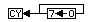Z: not affected
C: set according to result
N: reset (0)
H: reset (0)

RLC r or (HL)
Rotate Left Circular. This instruction rotates either register r of the byte located at the address in HL left one bit, placing bit 7 at bit 0 AND in the Carry flag.Z: set according to result
C: set according to result
N: reset (0)
H: reset (0)

RLA
Rotate Left Accumulator. This instruction rotates A left one bit, placing bit 7 into the Carry flag and the contents of the Carry flag into bit 0 of A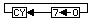Z: not affected
C: set according to result
N: reset (0)
H: reset (0)

RL r or (HL)
Rotate Left. This instruction rotates either register r or the byte located at the address in HL left one bit, placing bit 7 into the Carry flag and the contents of the Carry flag into bit 0 of AZ: set according to result
C: set according to result
N: reset (0)
H: reset (0)

RRCA
Rotate Right Circular Accumulator. This instruction rotates A right one bit, placing bit 0 at bit 7 AND in the Carry flag.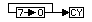Z: not affected
C: set according to result
N: reset (0)
H: reset (0)

RRC r or (HL)
Rotate Right Circular. This instruction rotates either register r of the byte located at the address in HL right one bit, placing bit 0 at bit 7 AND in the Carry flag.Z: set according to result
C: set according to result
N: reset (0)
H: reset (0)

RRA
Rotate Right Accumulator. This instruction rotates A right one bit, placing bit 0 into the Carry flag and the contents of the Carry flag into bit 7 of A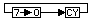Z: not affected
C: set according to result
N: reset (0)
H: reset (0)

RR r or (HL)
Rotate Right. This instruction rotates either register r or the byte located at the address in HL right one bit, placing bit 0 into the Carry flag and the contents of the Carry flag into bit 7 of AZ: set according to result
C: set according to result
N: reset (0)
H: reset (0)

SLA r or (HL)
Shift Left Arithmetically. This instruction shifts either register r or the byte located at the address in HL left one bit, placing 0 into bit 0, and placing bit 7 into the Carry flag.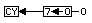Z: set according to result
C: set according to result
N: reset (0)
H: reset (0)

SRA r or (HL)
Shift Right Arithmetically. This instruction shifts either register r or the byte located at the address in HL right one bit, placing bit 0 into the Carry flag, and leaving bit 7 untouched.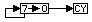Z: set according to result
C: set according to result
N: reset (0)
H: reset (0)

SRL r or (HL)
Shift Right Logically. This instruction shifts either register r or the byte located at the address in HL right one bit, placing 0 into bit 7, and placing bit 0 into the Carry flag.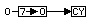Z: set according to result
C: set according to result
N: reset (0)
H: reset (0)

NOTE: there is no SLL instruction, seeing as every other type of shift has both left and right versions. The reason for this is that SHIFTING LEFT LOGICALLY ends up being identical to SHIFTING LEFT ARITHMETICALLY. Go figure.

### Bit Manipulation

Ok, so now that we know how to move whole registers around, what happens if we want to change a single bit or test the contents of a bit? Well that's where the instructions BIT, SET, and RES come in.

BIT b, r
BIT b, (HL)

Tests bit b in register r or the byte addressed in HL. Basically the specified bit gets copied to the Z flag AND INVERTED. Why inverted? Because the Z flag is set when a result is ZERO, that's why. =)
Flags affected are:
Z: set according to result
C: no effect
N: reset (0)
H: set (1)

SET b, r
SET b, (HL)

Sets (1) bit b in register r or the byte addressed in HL.
Flags affected are:
Z: no effect
C: no effect
N: no effect
H: no effect

RES b, r
RES b, (HL)

Resets (0) bit b in register r or the byte addressed in HL.
Flags affected are:
Z: no effect
C: no effect
N: no effect
H: no effect

Well that's about it for this lesson, that was alot of stuff to digest.

For all the specific limitations and abilities when using these opcodes, see the instruction set reference guide also on my webpage.